# Survival Analysis: Comparison of Two Survival Curves – Lachin

Hypothesis: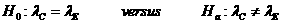Data Input: (Help) (Example)

 Input Results α β δ MS N QC ND QE T0 T-T0

Note:

 Variables Descriptions α Significance level 1-β Power of the test λC Hazard rate of control group λE Hazard rate of experimental group δ Minimum hazards ratio,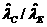, the ratio of two estimated hazard rates MS Control median survival (month) QC Proportion in control group QE Proportion in experimental group T0 Accrual duration (month) T-T0 Follow-up duration (month) N Sample size ND Total number of deaths

Help Aids

Application:  The determination of the number of patients needed in a prospective comparison of survival curves, when the control group patients have already been followed for some period, following hypotheses are usually used:Procedure:

1. Enter

a)    value of α, the probability of type I error

b)    value of β, the probability of type II error, or (1-power) of the test

c)    value of δ, the minimum hazards ratio, it is specified in alternate hypothesis

d)   value of MS, the control median survival (year), it is estimated from existing control data

e)    value of QC, the proportion of patient in control group compared with experimental group

f)    value of QE, the proportion of patient in experimental group compared with control group, where QC + QE = 1

g)   value of T0, accrual duration (year), the length of time to recruit patients for study.

h)   value of T-T0, follow-up duration (year), the length of study time of all recruited patients to the end of study T

1. Click the button “Calculate” to obtain the sample size of patients in the experimental arm and the total number of deaths at the end of study

Assumption:

1.      Time to survival is exponential distributed with hazard rate λ.

Formula:

Consider that there are two independent groups of sizes nC and nE all followed to the terminal event where time t is measured from the time of entry into the study.

Under null hypothesis,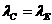, test statistic is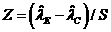, where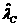and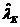are the estimated hazard rates,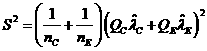, and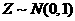For the determination of sample size and power one specified the minimal relevant difference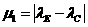, which yield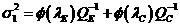. Under the null hypothesis,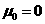and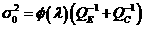where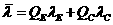.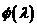is defined as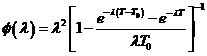The sample size to achieve specified significance level and power is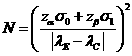, where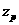is the upper 100(1-p) percentile of the standard normal distribution.

Here the calculator uses two-sided test. α of the above equation is replaced by α/2.

Notations:

α:         The probability of type I error (significance level) is the probability of rejecting the true null hypothesis.

β:         The probability of type II error (1 – power of the test) is the probability of not rejecting the false null hypothesis.

Example:

Consider that a clinical trial is to be conducted for a disease with moderate levels of mortality with hazard rare λ=0.30, yielding 50% survivors after 2.3 years (MS=2.3). Suppose that with treatment we are interested in a reduction in hazard to λ=0.20, i.e., an increase in survival to 64% at 2.3 years (δ=0.3/0.2=1.5). With equal-sized group (QC=QE=0.5), significance level test (α =0.05) with power 90% power (β=0.1), and assume that recruitment was to be terminated after 3 years of a 5-year study (T=5, T0=3, T-T0=2), then the required sample size is approximate 461 (N=461) and the total number of deaths is 264 (ND=461).

Reference: Lachin (1981) Controlled Clinical Trials 2: 93-113